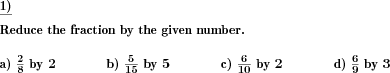Custom math worksheets at your fingertips# Details for problem "Reduce fractions by"

Quickname: 5814

Elementary School, Primary School, Junior High School, Middle School, High School.

## Summary

Fractions are to be reduced by a given number

## Example## Description

A fraction is given, and with it, a whole positive number. The second number is a factor of both the nominator and the denominator of the fraction. In fact, it is the greatest common factor of them, also called the greatest common divisor (GCD). The fraction has to be reduced by that number. The result will be a fraction with the same value as the original fraction, but it will be reduced to lowest terms.

The number range from which both numerator and denominator are randomly selected can be chosen.

In each problem, a single fraction is to be reduced. The number of problems and thus the number of fractions to be reduced can be specified.

Download free printable worksheets for this math problem here. The worksheet contains the problems only, the solution sheet includes the answers. Just click on the respective link.

•Worksheet 1Solution sheet with answers
•Worksheet 2Solution sheet with answers
•Worksheet 3Solution sheet with answers

If you can not see the solution sheets for download, they may be filtered out by an ad blocker that you may have installed. If this is the case, please allow ads for this page and reload the page. The solution sheets will then reappear.

• Do these sample worksheets do not really fit?
• Do you need more math worksheets, with a different level of difficulty?
• Would you like to combine different problems on a worksheet and adjust them to your needs?
• As a teacher, you can put together your own worksheets using the automatically generated math problems provided.
With a free initial credit, you can start creating your own math worksheets in a few minutes.

You can try it for free! Register here, to create custom worksheets now!

## Customization options for this problem

Parameter
Possible values
Number of problems
1, 2, 3, 4, 5, 6, 7, 8, 9, 10
Number range
20, 30, 40, 50, 100, 200, 500, 1000, 10000, 100000

## Similar problems

Remark
Description
Similar, reduction ot fractions by given numbers
Fractions are to be expanded by a given number
With no number given, reduce to lowest terms
A fraction has to be reduced to lowest terms.